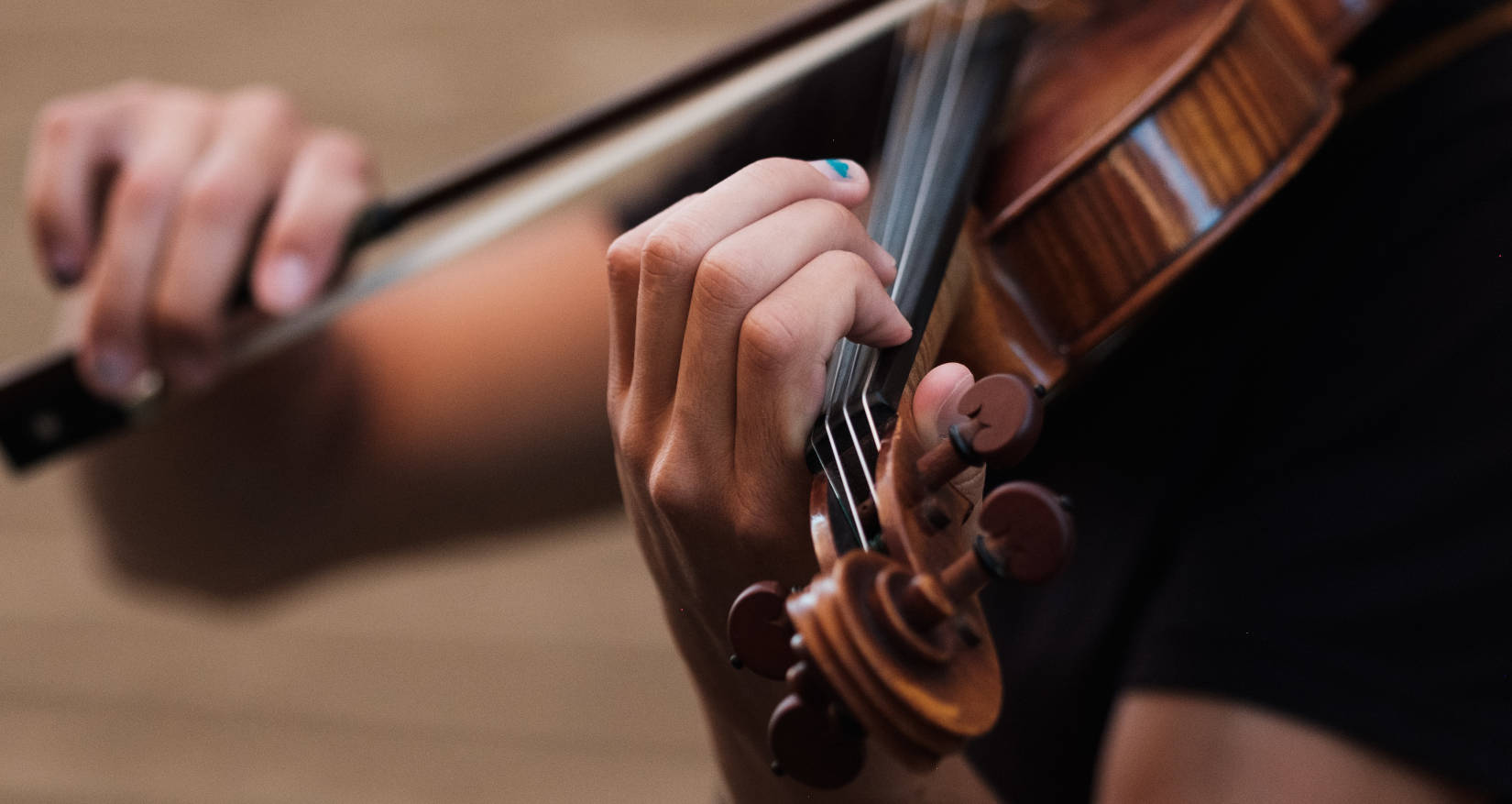# Calculating the Exact Finger Positions for a String Instrument

· Timo Denk

Playing string instruments like violin, viola, cello, or double bass requires the musician to place their finger at specific positions on the instrument’s fingerboard to play the desired tones. The musicians memorize the approximate finger positions and perform very quick, ideally imperceptible corrections once the tone is audible, using their relative hearing.

This blog post takes on the theoretical exercise of mathematically determining the exact finger positions using music theory and high school physics. It is worth pointing out that these computations are of little applied relevance, as the finger placement is ultimately done by hearing and not by seeing or through measurement.Before diving into the equations, let’s note down the problem statement once more. Above is a violin shown from the side. When playing its, say, A string, one will hear a tone at 440 Hz. The next tone on the chromatic scale is the A♯. What we want to calculate is the exact position at which the finger needs to push down the A string onto the fingerboard in order for the string to oscillate at the frequency of the A♯. And the same for B, C, C♯, …, as well as the other strings.

Let $f_\text{open}$ denote the frequency at which the open string oscillates. This depends on how it is tuned. For the violin the standard tuning is $f_\text{G}=196\text{ Hz}$ for the G string, $f_\text{D}\approx293.68\text{ Hz}$ for the D string, $f_\text{A}=440\text{ Hz}$ for the A string, and $f_\text{E}\approx659.28\text{ Hz}$ for the E string. The length of an open string depends on the violin. For Antonio Stradivari’s “Messiah” violin from 1716 – a reference for many later built instruments (and just by the way an instrument that has its own Wikipedia article) – the length of an open string is $l_\text{open}=0.328\text{ m}$ (source). Note that this is not the length of the entire string, but the vibrating length, i.e., the distance between nut and bridge.

We look for a function $o_s(n)$ which returns the offset from the nut at which we have to place our finger in order to play the $n$th tone of the chromatic scale on the particular string $s$. On the A string ($s=\text{A}$) the tone $n=0$ would be the A, i.e., the open string. The subsequent tones are A♯ at $n=1$, B at $n=2$, C at $n=3$, and so on.

We will now look at the A string-specific case, i.e., $o_\text{A}(n)$, and generalize to any string on any instrument (!) later. Recall that the frequency of the open string is $f_\text{A}=440\text{ Hz}$ and its length is $l_\text{open}=0.328\text{ m}$. This lets us infer the string’s specific velocity. It is defined according to Mersenne’s laws as $$v_\text{A}:=2f_\text{A}l_\text{open}=288.64\frac{\text{m}}{\text{s}},.$$ It tells us that waves propagate through the string at almost the speed of sound in the air. For the much thicker G string this velocity is lower, namely $v_\text{G}=128.58\frac{\text{m}}{\text{s}}$.

Given the A string’s velocity $v_\text{A}$ we can define a function mapping any frequency $f\in[f_\text{A},\infty)$ to the needed string length which resonates at that $f$: $$l_\text{A}(f):=\frac{v_\text{A}}{2f}=\frac{288.64\frac{\text{m}}{\text{s}}}{2f},.$$ In other words: This function tells us to which length one needs to shorten the A string in order to have it oscillate at frequency $f$.

In order to define $o_\text{A}(n)$ we need to map a tone $n$ to its frequency. The relationship is defined as $$f_\text{A}(n):=f_\text{A}\times2^{\frac{n}{12}},.$$ When plugging in some values it is apparent that this exponential relationship makes sense, e.g., $f_\text{A}(0)=440\text{ Hz}$ and for $n=12$, which is 12 halftone steps or one octave higher, $f_\text{A}(12)=880\text{ Hz}$, the next higher A.

Bringing the pieces together we can define \require{cancel} \begin{align}o_\text{A}(n) :=&l_\text{open}-l_\text{A}\left(f_\text{A}(n)\right)\\ =&l_\text{open}-\frac{v_\text{A}}{2f_\text{A}\times2^{\frac{n}{12}}}\\ =&l_\text{open}-\frac{\bcancel{2f_\text{A}}l_\text{open}}{\bcancel{2f_\text{A}}\times2^{\frac{n}{12}}},. \end{align}The reshaping shows that there is actually no dependence on the open string frequency whatsoever. The distances between the tones are the same for any string. In other words: $o_\text{A}(n)$ is the same as $o_\text{G}(n)$ and any other $o_s(n)$:
\begin{align} o(n)=&l_\text{open}-\frac{l_\text{open}}{2^{\frac{n}{12}}}\\ =&0.328\text{ m}-\frac{0.328\text{ m}}{2^{\frac{n}{12}}},.\end{align} Even more generally, one can say that for every halftone step up, one needs to shorten the string to $\sqrt{0.5}\approx94.39%$ of its length. Let’s look at some values of $o(n)$:

Halftone Finger distance from the nut G string D string A string E string
$n=0$ $0.00\text{ cm}$ (open string) G D A E
$n=1$ $1.84\text{ cm}$ G♯ D♯ A♯ F
$n=2$ $3.58\text{ cm}$ (1st pos.) A E B F♯
$n=3$ $5.22\text{ cm}$ A♯ F C G
$n=4$ $6.77\text{ cm}$ (2nd pos.) B F♯ C♯ G♯
$n=5$ $8.23\text{ cm}$ (3rd pos.) C G D A
$n=6$ $9.61\text{ cm}$ C♯ G♯ D♯ A♯
$n=7$ $10.91\text{ cm}$ D A E B
$\vdots$ $\vdots$ $\vdots$ $\vdots$ $\vdots$ $\vdots$
$n=29$ $26.66\text{ cm}$ C G D ASide view onto a violin where the exact finger positions are marked, each a halftone step apart. It can be nicely seen how the distances between two notes shrink. The figure is a remix of an SVG from here, created using Sketch.

And lastly, here is a table listing the distances for the four instruments of the strings family:

Halftone Violin $l_\text{open}=0.328\text{ m}$ Viola $l_\text{open}=0.370\text{ m}$ Cello $l_\text{open}=0.690\text{ m}$ Double bass $l_\text{open}=1.060\text{ m}$
$n=0$ $0.0\text{ cm}$ $0.0\text{ cm}$ $0.0\text{ cm}$ $0.0\text{ cm}$
$n=1$ $1.84\text{ cm}$ $2.08\text{ cm}$ $3.87\text{ cm}$ $5.95\text{ cm}$
$n=2$ $3.58\text{ cm}$ $4.04\text{ cm}$ $7.53\text{ cm}$ $11.56\text{ cm}$
$n=3$ $5.22\text{ cm}$ $5.89\text{ cm}$ $10.98\text{ cm}$ $16.86\text{ cm}$
$n=4$ $6.77\text{ cm}$ $7.63\text{ cm}$ $14.23\text{ cm}$ $21.87\text{ cm}$
$n=5$ $8.23\text{ cm}$ $9.28\text{ cm}$ $17.31\text{ cm}$ $26.59\text{ cm}$
$n=6$ $9.61\text{ cm}$ $10.84\text{ cm}$ $20.21\text{ cm}$ $31.05\text{ cm}$
$n=7$ $10.91\text{ cm}$ $12.31\text{ cm}$ $22.95\text{ cm}$ $35.25\text{ cm}$

The featured image of this blog is by Joel Wyncott showing a violinist placing a finger on the G string in second position to play an A.